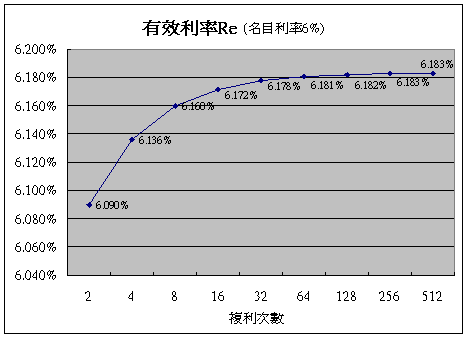﻿

# 每月複利一次的有效利率

FV = A*(1 + r)m ；A = 本金，r = 每期利率，m = 期數

FV = A*(1 + Rn / 12)12，利息所得是將12月後的終值減去期初本金A：

= FV – A
= A*(1 + Rn / 12)12 – A
= A * ((1 + Rn / 12)12 – 1)

= 利息 / 本金
= A * ((1 + Rn / 12)12 – 1) / A
= ((1 + Rn / 12)12 – 1

Re = (1 + R/12)12 – 1
= (1+5%/12)^12 -1
= 5.1162%

# 有效利率之公式

Re = (1 + Rn / m)m – 1，m 等於每年複利的次數

 複利期間 每年複利 次數(m) 有效利率(Re) 公式 有效利率(Re) 範例 名目利率Rn = 6% 季複利 4 =(1 + Rn / 4)4 – 1 =(1+6%/4)^4-1 = 6.136% 月複利 12 =(1 + Rn / 12)12 – 1 =(1+6%/12)^12-1 = 6.168% 日複利 365 =(1 + Rn / 365)365 – 1 =(1+6%/365)^365-1 = 6.183%# 結論

﻿

■ 本網站內容儘可能精確完整，但不保證無誤。若做為投資依據，風險請自行斟酌 ，本網站不負賠償之責任。
■ 網站所有資料均為版權所有，非經書面允許請勿轉載或使用。